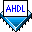## Predefined Evaluated Functions

 See AlsoThe following predefined evaluated functions are built into AHDL; that is, no Define Statements are needed to use them:

• The `USED` function can be used to test whether a port has been used, for example, in an If Generate Statement or a Parameters Statement. `USED` takes the port name as input and returns a value of `FALSE` if the port is not used.

• The `CEIL` function specifies the ceiling of a real number, which is the smallest integer that is equal to or greater than the real number. Although this operation applies to all arithmetic expressions, it is meaningful only for `LOG2` and `DIV` operations in which the result can be a real number.

• The `FLOOR` function specifies the floor of a real number, which is the largest integer that is equal to or less than the real number. Although this operation applies to all arithmetic expressions, it is meaningful only for `LOG2` and `DIV` operations in which the result can be a real number.

You can use these predefined evaluated functions in arithmetic expressions only; you cannot use evaluated functions in Boolean expressions.